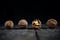# P versus NP Problem

P versus NP is a major unsolved problem in computer science, specifically in computability theory and computational complexity theory. The solution also will have profound implications in the fundamental understanding of mathematics, cryptography, artificial intelligence, life sciences, philosophy, economics and many other fields.
It is one of the seven millenium problems selected by Clay Mathematics Institute.Photo by Christian Fregnan on Unsplash

## Introduction

So we humans need to describe them how to solve a given problem, basically the ‘which and how’ about the computations.
The description has to be ‘unambiguous’ as it has to be followed by something which can not derive any sense out of ambiguity.

This unambiguous description about how to solve a given problem is called an “Algorithm”.
A computational problem always has some input data, and the solution is called the output data. An algorithm takes as input the input data of the problem and produces an output, which is the solution.

In order for an algorithm to reach to the solution, it needs ‘resources’, which can be anything the algorithm needs other than the input data.
For example; time needed to reach to solution, space needed to store intermediate computation data etc. can be called resources.

The input data of the problem can keep changing, for example; ‘how to multiply two numbers’ is a problem, but the numbers themselves can be anything and can change from one instance of the problem to another.
Also the numbers can be of any given size, this measurement of input data is called “input-size”.

Generally, resources required by an algorithm increase with the increase in input-size.
This growth in resource requirement with respect to the growth in input-size is called “asymptotic growth” and is represented as a ‘function of input-size’.

If the asymptotic growth of an algorithm can be represented as a polynomial function, then it’s called a “polynomial-time” algorithm and the problem is called to be solvable in polynomial-time.

Sometimes it’s possible to break a problem in terms of another problem.
For example, the problem of multiplication can be broken into problem of addition.
So if one figures out how to perform addition, then multiplication can be performed by performing repetitive addition.
This conversion of one problem into another is called “reduction”.
If both the time required in conversion and number of repetitions is polynomial, then the reduction is called “polynomial-time reduction”.

## Computational Complexity ClassesPhoto by Elijah O'Donnell on Unsplash

P is a set of problems which can be solved in polynomial-time.

NP is a set of problems which may or may not be solved on polynomial-time.
But if one has the solution, the correctness of the solution can be verified in polynomial-time.
Intuitively, all problems in P also belong to NP, as solutions of P can be verified in polynomial-time just by solving them.

NP-Hard is a set of problems to whom any problem in NP can be reduced to in polynomial-time.
There are no known polynomial-time algorithms to solve these problems. Also, some of these may not even be verifiable in polynomial-time, meaning these may or may not belong to NP.

NP-Complete is a set of problems which belong to both NP-Hard and NP.
Meaning, these are NP-Hard problems whose solution can be verified in polynomial-time.
NP-Complete is an intersection of sets NP and NP-Hard.

The classes in increasing order of complexity:
P < NP < NP-C < NP-H

# The ProblemPhoto by Olav Ahrens Røtne on Unsplash

It asks whether every problem whose solution can be verified in polynomial-time (NP) can also be solved in polynomial-time (P).
Meaning whether or not P = NP.

In other words, it asks whether there exists a polynomial-time algorithm for any of the NP-Complete problems.

## Consequences

As a philosophical consequence, it will change the picture of reality, where in solving a problem and verifying the solution would mean the same thing!
This will have a huge deal of implications on how we understand the world and reality.